Conge 精进

2019-02-20

# 01 - Technical versus fundamental analysisTwo broad categories of approaches to use for choosing stocks to buy or sell: Fundamental analysis and Technical analysis

Fundamental analysis: looking at aspects of a company to estimate its value and look for the price < value situations.

• earnings, dividends, cash flow, book value

Technical analysis: Looking for patterns or trends in a stock’s price.

Time: 00:00:36

# 02 - Characteristics

Characteristics of technical analysis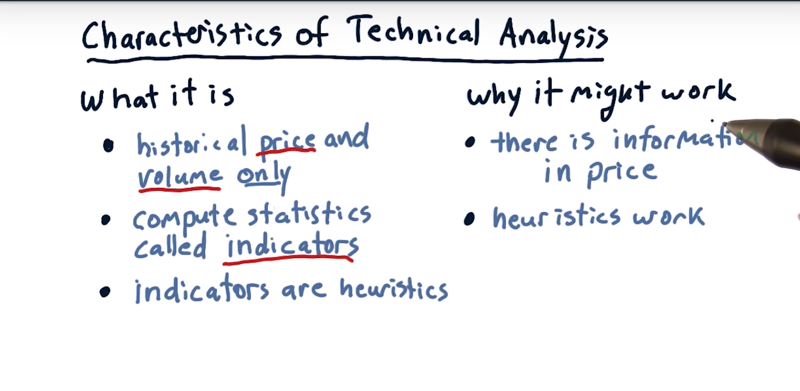1. it looks only at price and volume.
2. compute statistics (or indicators) on this time series
• Indicators are heuristics that may hint at a buy or sell opportunity.

Criticism of the technical approach

• it’s not an appropriate method for investing, because it’s not considering the value of the companies.
• trading approach as opposed to an investing approach.

Reasons to believe technical analysis

• there is indeed information in price and information in a price change.
• Stock price and price change reflects sentiments of buyers and sellers
• in other domains of artificial intelligence, heuristics can work, and they work frequently.

Time: 00:01:40

# 03 - Quiz: Potential indicators

Look at each one of these four factors and fill in the box, T for technical or F for fundamental.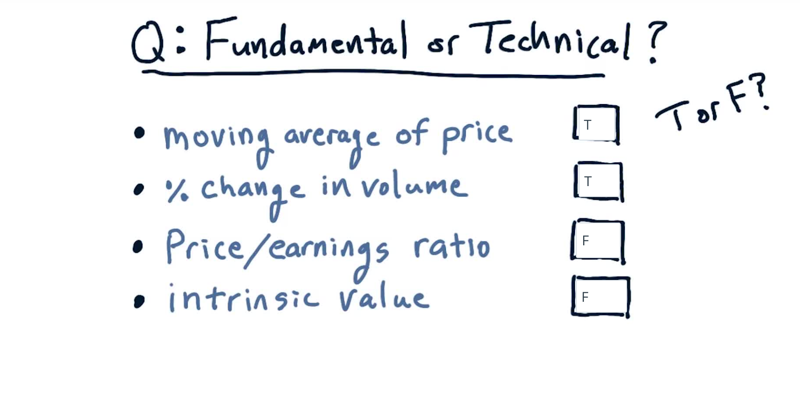• the moving average of price is only using price [technical.]
• Percent change in volume is only using volume [technical].
• price/earnings, earning is a fundamental factor, which makes the whole [fundamental].
• intrinsic value is based on dividends [a fundamenta]

Time: 00:00:41

# 04 - When is technical analysis valuable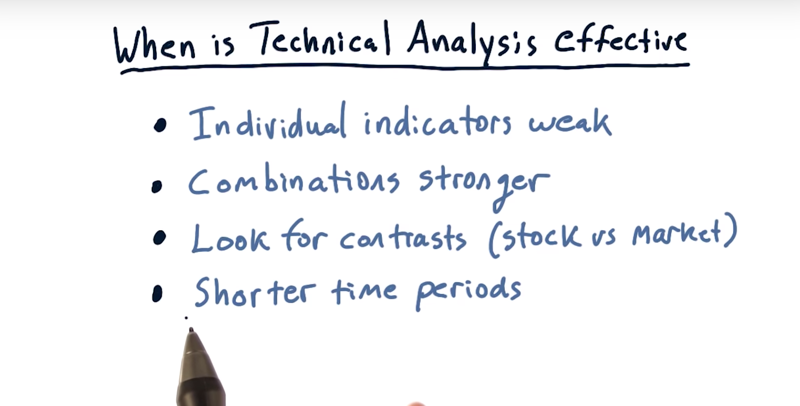1. individual indicators, by themselves, are weakly predictive. And the more people following a particular approach, the less value is realized
2. combining multiple indicators adds value. Combinations of three to five different indicators, in a machine learning context, provide a much stronger predictive system
3. contrasts. If certain stocks are behaving differently than the market, then they are worth a further look.
4. technical analysis generally works better over shorter time periods.

Time: 00:01:38

# 05 - When is technical analysis valuable part 2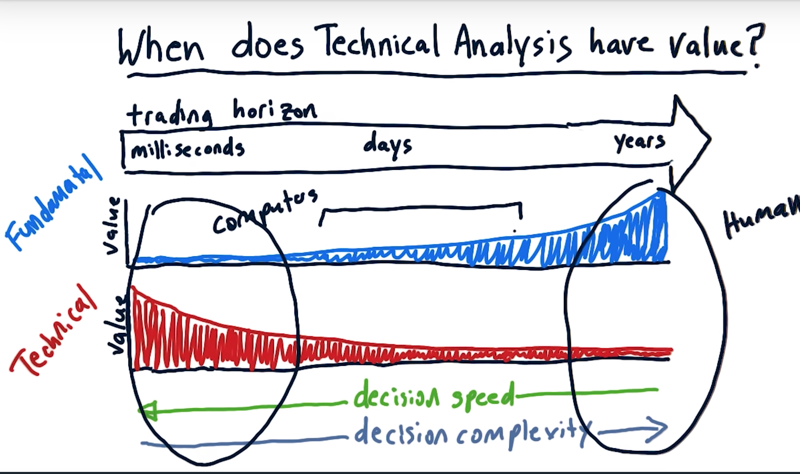For fundamental factors: Over long periods of time, fundamental factors provide a lot of value.

• For trading machines on the stock exchange, the order book or momentum, etc really matter, and the fundamental factors really have low value.
• fundamental factors over long periods of time may have significant value.
• over a period of days, Fundamental factors do have value.

for technical factors.

• over long terms technical analysis is not so valuable.
• technical factors potentially have high value over very short periods of time.

decision complexity

• we have to be able to make the decisions really, really fast if we’re trading at the millisecond level.
• at this very high-frequency trading, the complexity of decisions is simple. And computers excel in this region of the chart.

• the high-frequency trading computer-driven hedge funds are short in the trading horizon.
• human investors
• we can take a long time to make a decision if we’re going to buy and hold for years.
• If taking a long time to make a decision, it is the best region for human investors.
• The insight-driven, human-based hedge funds are operating over a long trading horizon.
• there is a middle where we often see humans and computers working together.

Time: 00:03:42

# 06 - A few indicators: Momentum

Three are some of the most common and most popular indicators: 1) momentum, 2( simple moving average, and 3) Bollinger Bands.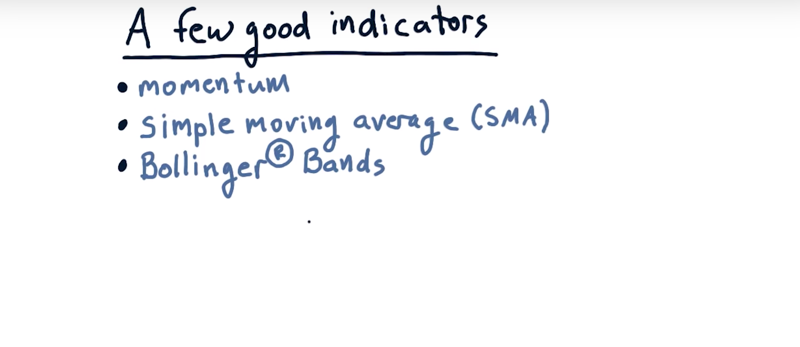momentum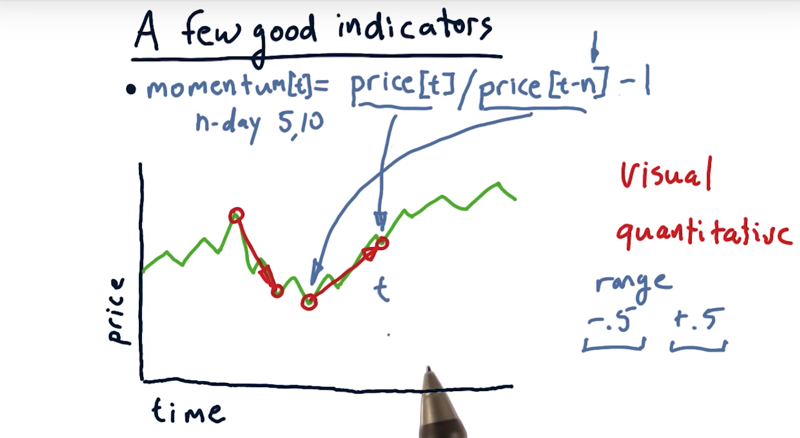Momentum is over some number of days how much has the price changed: positive momentum and negative momentum

• The steepness of that line is the strength of the momentum, either positive or negative.

• convert numbers
momentum, N days of momentum.

• the pseudo code for how we compute momentum on a particular day. `momentum[t] = price[t]/price[t - n] -1`, n is the number of days between two time point.

Time: 00:02:42

# 07 - A few indicators: Simple moving average (SMA).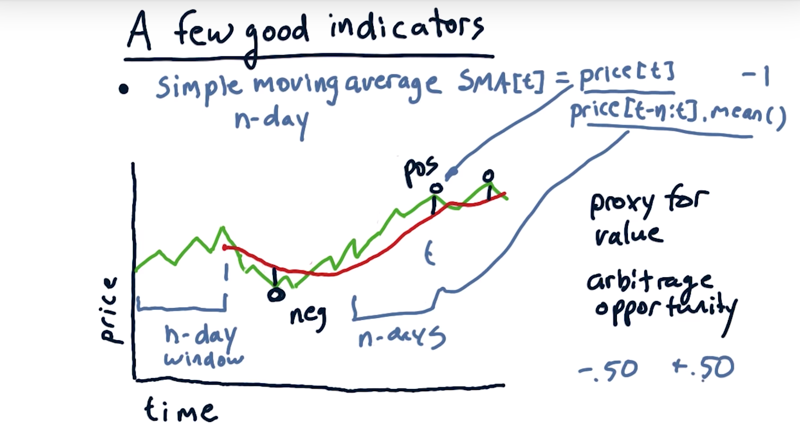• SMA for today is simply the average of the values over this look back window.
• the SMA looks essentially like a smoothed value of the price chart as it moves around.
• SMA lags the movement.

two different ways that technicians use simple moving average

1. look for where the current price crosses through the simple moving average.
• combine that with momentum: the price has strong momentum, and it’s crossing through that simple moving average, that can be a signal.
• strong momentum crossing those lines, then again, that can be a trading signal.
1. simple moving average is as a proxy for underlying value.
• The average price might represent the true value of the company.
• we should expect that the price is eventually going to regress to that average. ( it’s an arbitrage opportunity)

quantify SMA

• compare the current price with the current simple moving average, and construct a ratio.
• `SMA[t] = price[n]/price[t-n:t].mean() -1`
• This value ranges from -50% to +50%.

Time: 00:03:40

# 08 - A few indicators Bollinger Bands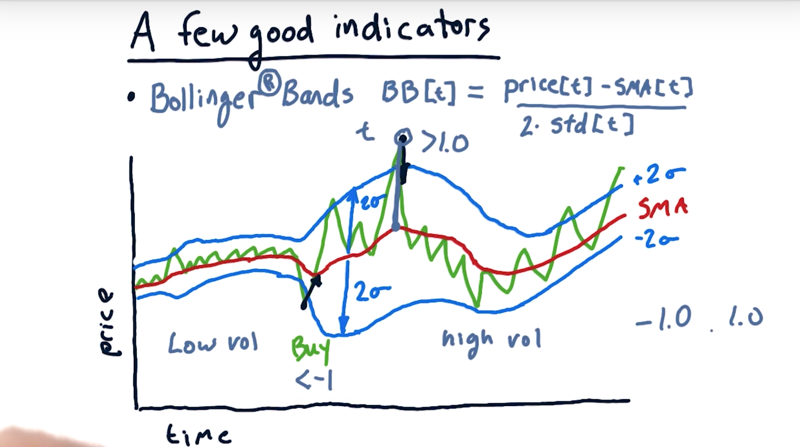how much of an excursion from the simple moving average should I use as a signal for a buy or sell?

• a fixed number is probably not the best way to go.
• John Bollinger observed that for low volatility, use a smaller excursion for that trigger. For high volatility, use a larger number.
• the Bollinger Bands: take this simple moving average, but let’s add a band above and below 2 std (standard deviations).

How might we use Bollinger Bands now for trading signals

• look for times where the price’s outside one of these Bollinger Bands and when it crosses to the inside it should be a trading signal.
• Moving from above the band and cross to the inside, selling signal
• Moving from below the band and cross to the inside, buying signal

How to calculate the Bollinger Band on a particular day t

• `BB[t] = (Price[t] -SMA[t]) / (2*std[t])`
• BB[t] = -1 or BB[t] = 1 are the crossing point.
• BB[t -1] > 1and BB[t] = 1 and BB[t+1] > 1, then Sell
• BB[t -1] < -1and BB[t] = -1 and BB[t+1] <- 1, then buy
• typically expect to BB[t] is between -1 and 1.
• occasionally, we’ll see excursions above and below those values.

Time: 00:03:46

# 09 - Buy or sell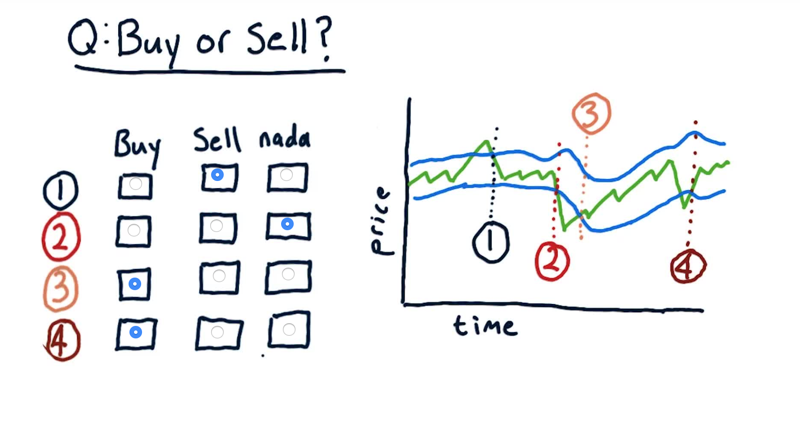In the four different times where the actual price crosses and upper or lower Bollinger band. Is it a buy signal, a sell signal or no signal at all?

The solution: is in the picture: remember, only when price moves into the Bollinger Band is a trading signal. Moving outside is not.

Time: 00:00:57

# 10 - Normalization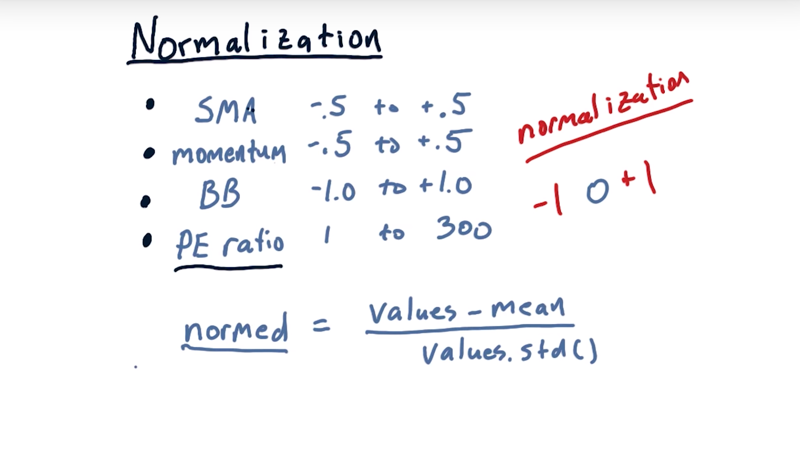• Simple moving average and momentum are bouned in [-.5, +.5] and Bollinger bands in [-1, 1].
• When used in a machine learner Bollinger Band factor would tend to overwhelm these other factors and [falsely] become the most important one.
• It might get even worse if we included fundamental factor like PE ratio ranges in [1, 300]

The solution: normalization.

Given values, the normalization works this way:

• `normalized_values = [values - mean(values)] / std(values)`
• normalized_values will range in [-1, 1] and have a mean of 0.

⚠️!!Normalize your data if use indicators in machine learning models!!

Time: 00:01:43

# 11 - Wrap up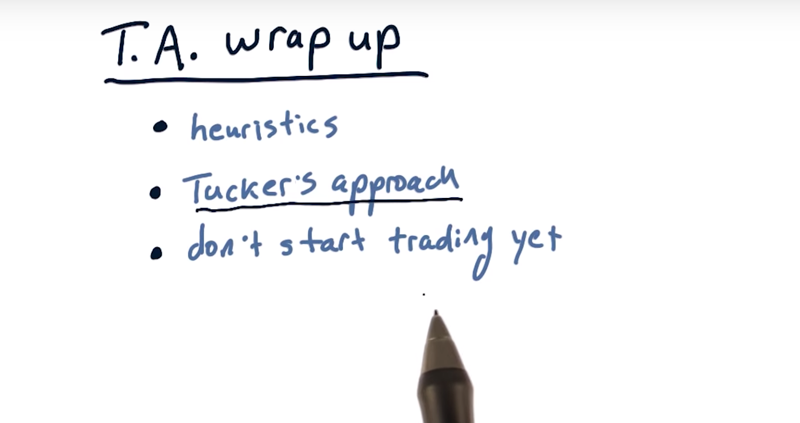1. technical indicators are really heuristics that represent how a statistical approach to previous prices and volume might suggest future price movement.

2. Tucker’s approach of using Bollinger Band.

``````2019-02-20 初稿